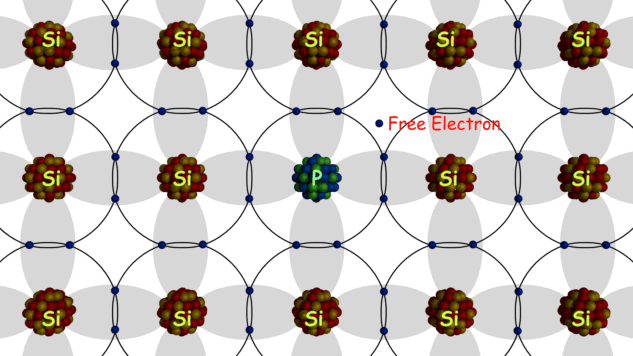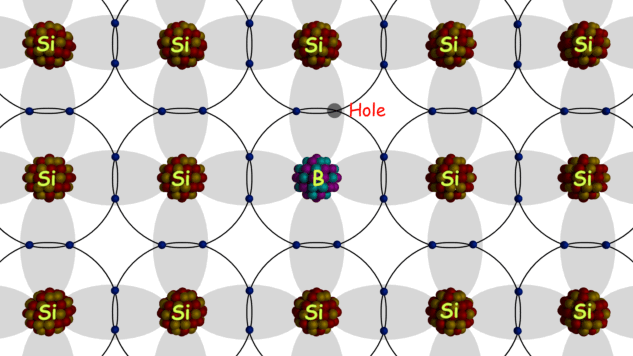# Donor and Acceptor Impurities in Semiconductor

When we add a small quantity of impurity in a semiconductor than the impurity contributes either free electrons or holes to the semiconductor. As a result, the conducting property of semiconductor changes. The process of changing the conductive property of semiconductor by adding impurities is known as doping. Suppose, we add any pentavalent impurity in any intrinsic semiconductor. The pentavalent impurities are those which have atoms with five (5) valence electrons. As soon as we add the impurity to the semiconductor, the impurity atoms will replace some of the semiconductor atoms in the crystal structure. Now four (4) of the five (5) valance electrons of impurity atom will involve in bonding with four neighbourhood semiconductor atoms, but the fifth one electron will not find any place to occupy.

This fifth electron of the impurity atom can be available as free electron or negative charge carrier even if a minimal amount of energy is applied. The energy required to detach the this fifth electron from its parent atom is in the range of 0.01 eV (electron volt) to 0.05 eV (electron volt). Antimony, Phosphorus, and Arsenic are usually and commercially used pentavalent impurities for a semiconductor. As pentavalent impurities contribute or donate electrons to the semiconductor, these are called donor impurities and similarly as these impurities contribute negative charge carriers in the semiconductor this we refer as n-type impurities. The semiconductor doped with n-type impurities is called n-type semiconductor.

## N Type Semiconductor

When we add n-type or donor impurities to the semiconductor, the width of the forbidden energy gap in the lattice structure is reduced. Due to addition of donor atoms, allowable energy levels are introduced a small distance below the conduction band as is shown in the figure below. These new allowable levels are discrete because the added impurity atoms are placed far apart and hence their interaction is small. In the germanium the discrete allowable energy level or forbidden energy gap is only 0.01 eV (electron volt) and that for silicon is 0.05 eV (electron volt) at room temperature. So it is predictable at room temperature the fifth electron of all donor atoms is in the conduction band. On the other hand, the increased number of negative charge carriers i.e. electrons in the semiconductor causes the decrease in some holes.

The number of holes per unit volume in an n-type semiconductor is even lower than that in the same unit volume of intrinsic semiconductor at the same temperature. This is because of excess electrons, and there will be the higher rate of recombination of electron-hole pairs than that in a pure or intrinsic semiconductor.## P Type Semiconductor

If instead of pentavalent impurity, a trivalent impurity is added to the intrinsic semiconductor, then instead of excess electrons there will be excess holes created in the crystal. Because when a trivalent impurity is added to the semiconductor crystal, the trivalent atoms will replace some of the tetravalent semiconductor atoms. The three (3) valance electrons of trivalent impurity atom will make the bond with three neighborhood semiconductor atoms. Hence, there will be the lack of an electron in one bond of the fourth neighboring semiconductor atom which contributes a whole to the crystal. Since trivalent impurities contribute excess holes to semiconductor crystal, and these holes can accept electrons, these impurities are referred as acceptor impurities. As the holes virtually carry positive charge, the said impurities are referred as positive – type or p type impurities and the semiconductor with p type impurities is called p-type semiconductor.When trivalent impurities are added to a semiconductor, a discrete energy level is created just above the valence band of the semiconductor. As the gap between valence energy level and the new discrete energy level created by impurity is quite less, the electron can easily migrate to the new higher energy level with the help of tiny amount of external energy. When an electron migrates to new energy level, it creates a vacancy in the valence band behind it, and this contributes hold to the semiconductor.

When we add an n-type impurity to the semiconductor, there will be excess electron is a crystal but it does not mean that there would not be any hole. Due to intrinsic nature of semiconductor at room temperature, there are always be some electron-holes pairs in the semiconductor. Due to an addition of n-type impurities, the electrons will be added to that electron-hole pairs and also the number of holes reduced excess recombination for excess electrons. Hence, the total number of negative charge carriers or free electrons will be more than that of holes in the n-type semiconductor. That is why in n-type semiconductor, the electrons are called majority charge carriers whereas poles are called minority charge carriers. Similarly in p-type semiconductor, holes are called the majority charge carriers and electrons are called minority charge carriers.

Want To Learn Faster? 🎓
Get electrical articles delivered to your inbox every week.
No credit card required—it’s 100% free.